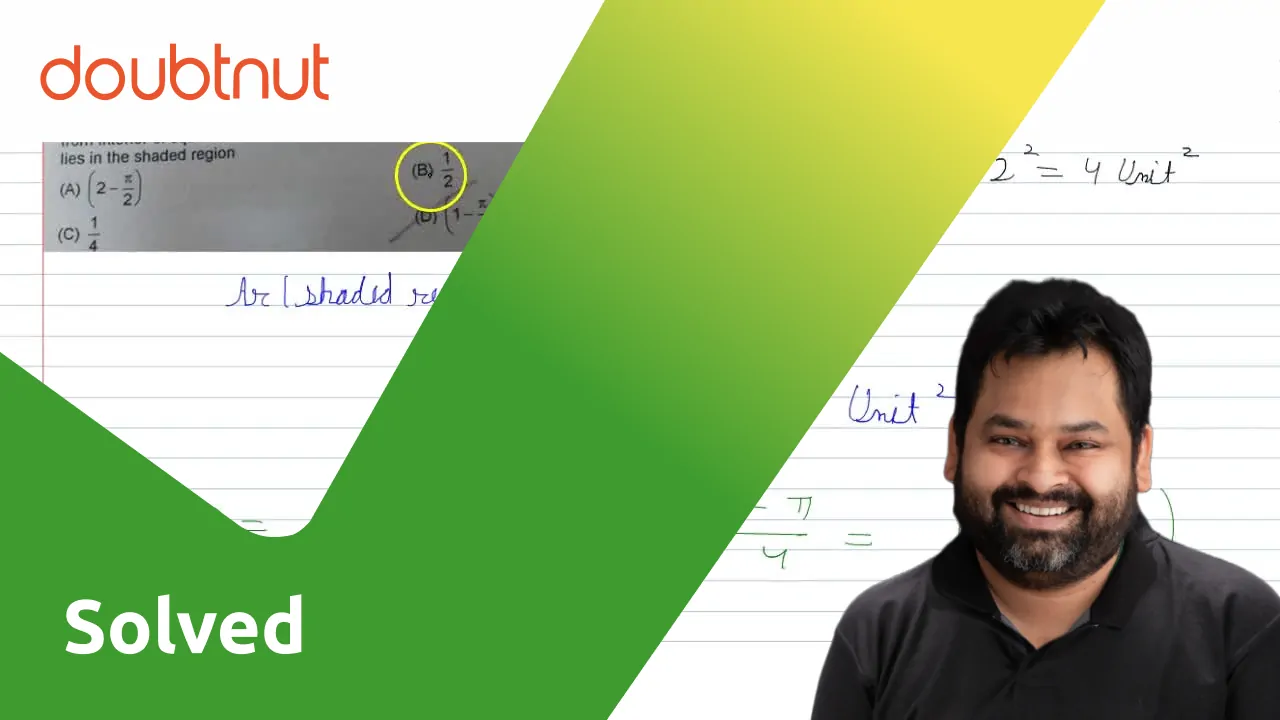Doubtnut App - Get 50 Lakh+ video solutions better than GoogleInstall Now

# In the given figure, A, B, C and D are centres of four circles that have a radius of 1 unit. If a point is selected at random from interior of square ABCD, then what is the probability that it lies in the shaded region

Text Solution
Verified by Experts

## Here, Side of the squareABCD=2∗1=2 units ∴ Area of square ABCD(A1)=22=4 square units Now, area of the shaded region (AS)=A1−4∗πr24 ⇒AS=4−π(1)2=4−π So, the required probability =ASA1=4−π4=1−π4.